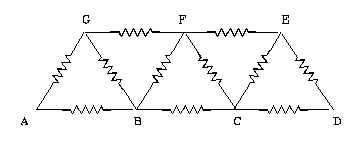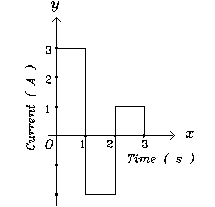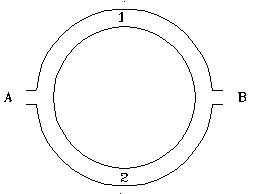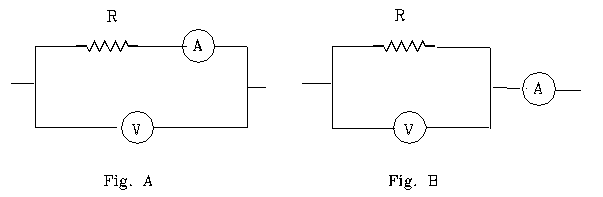PHYSICS

 Time: ONE Hour Max. Marks: 60

• Answers must be written either in English or the medium of instruction of the candidate in high school.
• Answer all the questions in the booklets provided for the purpose.
• There will be no negative marking.
• The relevant working in arriving at an answer has to be shown wherever required. However if the answer can be arrived at without detailed working, write only the answer
• Use of calculators or graph papers is not permitted.
• Questions in Part A carry 5 marks each and questions in part B carry 3 marks each.

Part A

(6x5=30 Marks)

1. Two small stones are tied at the ends of a rope of length 5 m . Imagine you are holding them, one in each hand, with the rope hanging loosely on the top floor balcony of a tall building. You release one stone at a certain moment and the other 0.5 s later along the same vertical line from the same position. The rope will be taut after a time of                           . Assume that as the rope gets taut, the velocities of the stones becomes equal to the average of the velocities of the stones just before the stone gets taut. The velocities of the stones 2 s later would be                         .

2. A concave mirror of focal length 25 cm and a convex lens of focal length 20 cm are arranged coaxially 85 cm apart. A point object is located on the principal axis of the system 30 cm from the convex lens. Rays of light after passing through the lens fall on the mirror and after reflection from the mirror pass through the lens again. These rays converge at a distance of                             from the lens after passing through it a second time.

 All Resistances are equal to 9 Ohm each. Equivalent resistance between B and C is _________________ and the equivalent resistance between A and D when the resistances between GF and FE are removed is _________________.1. A cylindrical wire of radius R is made of two different materials. The inner portion of radius R/2 is made of a material of resistivity r and the outer annular portion of thickness R/2 is made of material of resistivity 2r . Resistance per unit length of this wire would be                            .

1. A boat moves down stream a certain distance in a river. If the river were not flowing the time taken would have been 2 hrs but with the river flowing the time taken is 1.5 hrs. The time taken to travel upstream the same distance would be                      .

 Current flowing through a wire of resistance 2 Ohm varies with time as shown. The amount of heat produced and the charge that crosses a given section of the wire in 3 s would be               and ________________.Part B

(10x3=30 Marks)

1. Imagine a man moving from north pole to the equator and from the equator to the south pole, moving along the same longitude. The magnitude of the displacement in the first and second parts are _____________ and ______________ and the total displacement is ____________.

2. A chain is lying as a heap on a table. One end of it is pulled vertically upward with a constant acceleration of 0.5 m/s2. The velocity of the mid point of the vertical portion when the upper end is at height of 0.64 m above the table is                          .

3. A carom striker hits a side of the carom board at some angle and rebounds. It then hits the adjacent side. The angle between the initial and final directions of motion is ____________. (Assume the angles made by the line of motion with the normal before and after collision are same for both the collisions)

 A thin circular tube of radius r has two openings at diametrically opposite ends. A source of sound of frequency n is located at A. Sound travels along the paths 1 and 2 to B. The temperatures of air in 1 is higher than the temperature of air in 2 and the corresponding speeds of sounds are v1 and v2 respectively. The number of extra waves contained in one path as compared to other is _________ _ . If a pulse of sound were to be emitted at A the time interval between the moments the pulse arrives at B along the two paths to B is                        .1. A sphere consists of inner portion of radius r made of a material of density 8 gm/cm3 , and an outer layer of thickness t made of a material of density 4 gm/cm3. This sphere floats in a liquid of density 10 gm/cm3 with half its volume submerged. The ratio r/t is equal to _____________________.

 The value of a resistance R can be determined by connecting it either as shown in Fig A or as shown in Fig B. When connected as in A the readings are 2 A and 100 V and when connected as in B the readings are 2.1 A and 98 V. The resistance would lie between ______________ and ______________. If the resistance of ammeter is 2 Ohm the value of R is                           .1. Sound wave of a frequency n travels in a certain gaseous medium with a velocity v. A second gaseous medium at same pressure as the first has twice greater density. The speed of sound of frequency 2n in this medium would be                          .

2. A radio active substance of half life period 2 hrs is being produced in a reactor. At the initial moment the mass of the substance is zero and production rate is 5 mg/s. The substance is produced for 10 s. Two hours later the mass of the radioactive substance would be nearly                .

3. A negatively charged particle moving at right angles to a magnetic field in the southeast direction experiences a vertical upward force. The force experienced by a positively charged particle moving in the northwest direction would be                          .

4. Energy released in a fusion reaction of two Deuterons is nearly 24 MeV. Energy released in the fission of U235 is nearly 200 MeV. The mass of Uranium that should undergo fission to release the same amount of energy as obtained by fusing nuclei contained in 1 kg Deuterons would be                    and the energy released is                       .